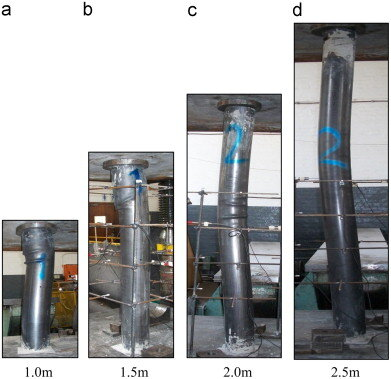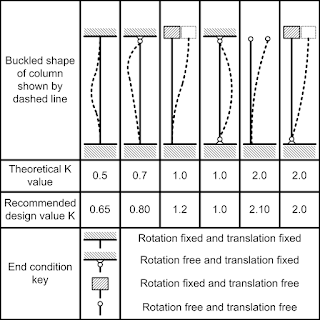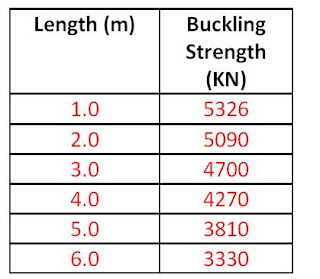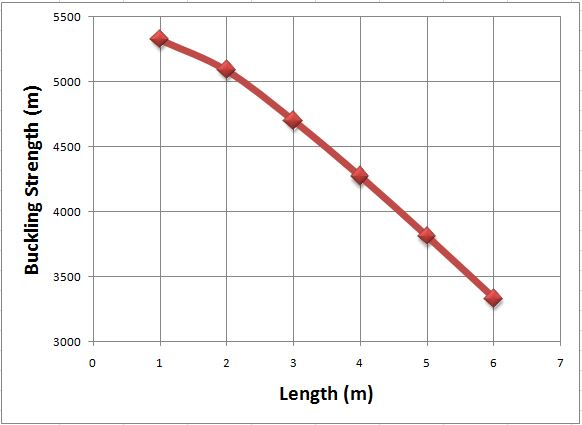Home Steel Structures Flexural Buckling of Columns

# Flexural Buckling of ColumnsWhen structural members are subjected to compressive forces, the members may fail before the compressive resistance (A.fy) is reached. This premature failure is usually caused by secondary bending effects such as imperfections, eccentricity of loading, asymmetry of the cross-section etc. In such cases, the failure mode is normally buckling.

This is unlike when the member is subjected to tensile forces, where the member will generally fail when the stress in the cross-section exceeds the ultimate strength of the material. Members subjected to tensile forces are inherently stable.

In essence for very short columns or members subjected to tensile forces, the stress is proportional to the load, and the failure load is equal to the yield stress times the area of the cross-section (A.fy). Commonly, compression members can be classified as short, intermediate, or slender depending on their slenderness ratio. Short elements will fail by crushing, slender elements will fail by excessive lateral deflection, while intermediate elements will fail by combination of both.

Types of Buckling
(1) Flexural buckling (Euler)
(2) Lateral-torsional buckling
(3) Torsional buckling
(4) Snap-through buckling
(5) Local plate buckling

In this post, we are going to focus on flexural buckling.

In the year 1757, Leonhard Euler developed a theoretical basis for analysis of premature failure due to buckling. The theory was based on the differential equation of elastic bending of of a pin-ended column, which related the applied bending moment to the curvature along the length of the column.

For a pin ended column, the critical Euler buckling load (n = 1.0) is given by;

PE = π2EI/L2Note that L in this case is the effective buckling length which depends on the buckling length between the pinned supports or the points of contraflexure for members with other boundary conditions.

Subsequently, Perry-Robertson formular was developed to take into the account the deficiencies of the Euler method. The formular evolved from the assumption that all practical imperfections could be represented by a hypothetical initial curvature of the column. In the UK, the Perry-Robertson formula was modified and is refereed to in BS 5950 as the Perry-Strut formular. As a matter of fact, it also forms the basis of flexural buckling in BS EN 1993 (Eurocode 3).

Solved Example
In the example below, we are going to show how the failure load of a column decreases with increase in effective length due to the phenomenon of buckling.

Let us investigate the load carrying capacity of UC 305 x 305 x 158 according to EC3. We will consider the ends of the column to be pinned, and we will start with an intial length of 1.0 m.

Properties of UC 305 × 305 × 158

D = 327.1 mm; B = 311.2 mm; tf = 25mm; tw = 15.8mm; r = 15.2mm; d = 246.7mm, b/tf = 6.22; d/tw = 15.6; Izz = 38800 cm4; Iyy = 12600 cm4; iy-y = 13.9cm; iz-z = 7.9 cm, A = 201 cm2Thickness of flange tf = 25mm.
Since tf  > 16mm, Design yield strength Fy = 265 N/mm2 (Table 3.1 EC3)

Section classification

ε = √(235/Fy ) = √(235/265) = 0.942

We can calculate the outstand of the flange (flange under compression)
C = (b – tw – 2r) / (2 ) = (327.1 – 15.8 -2(15.2)) / (2 ) = 140.45mm.
We can then verify that C/tf = 140.45/25 = 5.618
5.618 < 9ε i.e. 5.618 < 8.478.

Therefore the flange is class 1 plastic

Web (Internal compression)
d/tw = 15.6 < 33ε so that 15.6 < 31.088. Therefore the web is also class 1 plastic

Resistance of the member to uniform compression (clause 6.2.4)
NC,Rd = (A.Fy)/γmo = (201 × 102 × 265) / 1.0 = 5326500 N = 5326.5 KN

Buckling resistance of member (clause 6.3.1)

Since member is pinned at both ends, critical buckling length is the same for all axis Lcr = 1000mm

Slenderness ratio ¯λ = Lcr/(ri λ1)
λ1 = 93.9ε = 93.9 × 0.942 = 88.454

In the major axis
(¯λy ) = 1000/(139 × 88.454) = 0.081
In the minor axis
(¯λz ) = 1000/(79 × 88.454) = 0.143

Check h/b ratio = 327.1/311.2 = 1.0510 < 1.2, and tf < 100 mm (Table 6.2 EN 1993-1-1:2005)

Therefore buckling curve b is appropriate for y-y axis, and buckling curve c for z-z axis. The imperfection factor for buckling curve b α = 0.34 and curve c = 0.49 (Table 6.1)

Φ = 0.5 [1 + α(¯λ – 0.2) + ¯λ2]

Φz = 0.5 [1 + 0.49 (0.143 – 0.2) + 0.1432 ] = 0.496
Φy = 0.5 [1 + 0.34 (0.081 – 0.2) + 0.0812 ] = 0.483

X = 1/(Φ  + √(Φ2 -¯λ2))
Xz = 1/( 0.496 + √(0.4962 – 0.1432)) = 1.02 > 1 (cannot be greater than 1, so take 1.0)
Xy = 1/( 0.483 + √(0.4832 – 0.0812)) = 1.04 > 1 (cannot be greater than 1, so take 1.0)

Therefore Nb,Rd = (Xz A.Fy)/γm1 = (1.0 × 201 × 102 × 265) / (1.0 ) = 5326500 N = 5326.5 KN

Nb,Rd = NC,Rd = 5326.5 KN

This tells us that the column is short, and that at length of 1.0 m, the column will fail by crushing. Buckling is not a significant mode of failure.

If we incrementally increase the length of the column by 1.0 m, and following the steps described above, we will arrive at the following results;The graph of the relationship is given below;Therefore, the strength of compression members reduce with increase in length, and that is why it is advisable to design your trusses in such a way that the longer members are in tension, and the shorter members in compression. It gives more economical results.

Thank you for visiting Structville today, and God bless.